# Determination of price and quantity supplied by price-discriminating monopolistic firm in the short run

Jump to: navigation, search

This article discusses a variation of the determination of price and quantity supplied by monopolistic firm in the short run where the firm is capable of price discrimination, i.e., charging different prices to different market segments. We discuss the case of perfect price discrimination, and then other cases.

## The general optimization principles

• The basic idea carries forth from determination of price and quantity supplied by monopolistic firm in the short run: an optimal choice (unless it's at an extreme quantity of production) occurs at a quantity of production where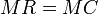$MR = MC$ (where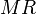$MR$ and$MC$ denote respectively the marginal revenue and marginal cost functions), with the marginal cost curve overtaking the marginal revenue curve.
• The main difference now is that the marginal revenue function is defined differently.

## Market with two segments

Consider a situation where the market is subdivided into two segments. The market demand curve is the sum of the demand curves for the two market segments. The segments are already available to the seller. The seller has to choose a price for each segment, plus a quantity of production that equals the total of the quantities that would be demanded by the segments at their respective prices.

### Extreme case

The following are equivalent:

• For the optimal choice, the seller chooses the same price for both market segments.
• For the optimal choice, the seller chooses the same price for both market segments as the optimal price would be if the seller couldn't practice price discrimination.
• Price discrimination based on this particular market segmentation does not increase the seller's profit.

This extreme case is quite rare.

### Optimization problem in the case of constant marginal cost

If the marginal cost curve is horizontal, then the production processes for the two submarkets can be treated separately, and the problem reduces to carrying out the determination of price and quantity supplied by monopolistic firm in the short run separately for the two markets.

### Optimization problem in the general case

In cases that the marginal cost curve is not horizontal, the problem cannot neatly be broken down into problems for the submarkets. This is because even though the good is being sold in two separate markets, it is being produced in a single factory, and in particular has a single combined marginal cost curve. The choice of how much to produce for one market affects the cost of production using the production capacity remaining for the other market.

To solve this problem, we need to perform an optimization for a function of two variables. Graphically, this works as follows. Consider a three-dimensional graph setup where two axes are quantity axes (for the quantities produced for the two submarkets) and the third axis is used to plot price-like quantities (such as marginal revenue and marginal cost).

#### Variable cost function

The variable cost function for this pair of quantities is defined as the marginal cost for the sum of the quantities. Geometrically, the variable cost surface is obtained as follows: consider the original marginal cost curve. Make lines in the quantity plane correspond to constant total quantity. Above each such line, the variable cost surface has constant height, equal to the variable cost for that total quantity.

Algebraically, if$Q_1$ and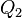$Q_2$ denote the quantities for the two market segments, and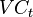$VC_t$ denotes the original marginal cost function for total quantity, then: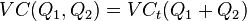$VC(Q_1,Q_2) = VC_t(Q_1 + Q_2)$

#### Revenue function

The revenue function for the pair of quantities is the sum of the marginal revenue functions for each quantity in its respective market segment. In other words, the revenue function is additively separable.

Algebraically, if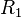$R_1$ and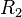$R_2$ denote the marginal revenues for the two market segments, the combined revenue is given as: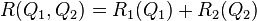$R(Q_1,Q_2) = R_1(Q_1) + R_2(Q_2)$

Recall that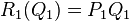$R_1(Q_1) = P_1Q_1$ where$P_1$ is the price corresponding to$Q_1$, and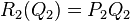$R_2(Q_2) = P_2Q_2$ where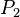$P_2$ is the price corresponding to$Q_2$. Therefore: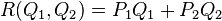$R(Q_1,Q_2) = P_1Q_1 + P_2Q_2$

#### Profit function

The profit function in this case is: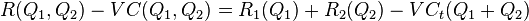$R(Q_1,Q_2) - VC(Q_1,Q_2) = R_1(Q_1) + R_2(Q_2) - VC_t(Q_1 + Q_2)$

This is a function of two variables, and can be optimized using the techniques for optimizing functions of two variables.

#### Optimal choice

Assuming a solution not at the boundary, and assuming differentiability, we obtain that the first-order partials with respect to both$Q_1$ and$Q_2$ are zero (see here for technical background). Explicitly, we obtain the condition: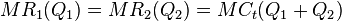$MR_1(Q_1) = MR_2(Q_2) = MC_t(Q_1 + Q_2)$

This does have the flavor of$MR = MC$, but with a difference: we want the marginal revenue from both markets to equal each other and to equal the marginal cost of combined production (rather than the marginal cost of producing for a particular market). As a sanity check, the analysis of the earlier case of constant marginal cost is a special case of this. As another sanity check, this is a system with two equations and two variables ($Q_1$ and$Q_2$).

We also need second-order conditions to ensure that this is a point of local maximum. Explicitly, in this case, the second-order condition would imply that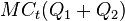$MC_t(Q_1 + Q_2)$ is overtaking both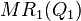$MR_1(Q_1)$ and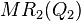$MR_2(Q_2)$. It would also imply a Hessian condition that is too complicated to explore here.

## Market with multiple segments

The general analysis of a market with two segments carries forth to multiple segments. Explicitly, the revenue function is additively separable across the markets, whereas the cost function is not, but rather depends on the total quantity produced. If the quantities produced in the$n$ market segments are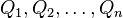$Q_1,Q_2,\dots,Q_n$, the marginal revenue functions are$MR_1, MR_2, \dots, MR_n$, and the marginal cost function for total quantity produced is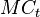$MC_t$ then the first-order condition is:$MR_1(Q_1) = MR_2(Q_2) = \dots = MR_n(Q_n) = MC_t(Q_1 + Q_2 + \dots + Q_n)$

This is a system of equations with$n$ equations and$n$ variables.

## Upper bound on the profit that can be gained through segmentation

Regardless of the level of segmentation, the following is a hard upper bound on the profit that a price-discriminating monopolistic firm can make:

• Plot the marginal cost curve and the market demand curve, and take the intersection where the marginal cost curve is rising and the market demand curve is falling.
• The area between the marginal cost curve and the market demand curve, with the quantity ranging from zero to the quantity at the point of intersection, is the upper bound on profit.